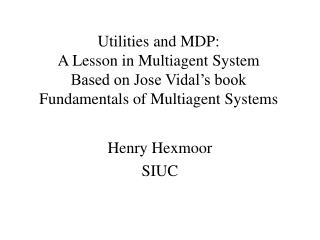# Henry Hexmoor SIUC - PowerPoint PPT PresentationDownload PresentationHenry Hexmoor SIUC

Download Presentation## Henry Hexmoor SIUC

- - - - - - - - - - - - - - - - - - - - - - - - - - - E N D - - - - - - - - - - - - - - - - - - - - - - - - - - -
##### Presentation Transcript

1. Utilities and MDP: A Lesson in MultiagentSystemBased on Jose Vidal’s bookFundamentals of Multiagent Systems Henry Hexmoor SIUC

2. Utility • Preferences are recorded as a utility function S is the set of observable states in the world ui is utility function R is real numbers • States of the world become ordered.

3. Properties of Utilites • Reflexive: ui(s) ≥ ui(s) • Transitive: If ui(a) ≥ ui(b) and ui(b) ≥ ui(c) then ui(a) ≥ ui(c). • Comparable: a,b either ui(a) ≥ ui(b) or ui(b) ≥ ui(a).

4. Selfish agents: • A rational agent is one that wants to maximize its utilities, but intends no harm. Agents Rational agents Selfish agents Rational, non-selfish agents

5. Utility is not money: • while utility represents an agent’s preferences it is not necessarily equated with money. In fact, the utility of money has been found to be roughly logarithmic.

6. Marginal Utility • Marginal utility is the utility gained from next event Example: getting A for an A student. versus A for an B student

7. Transition function Transition function is represented as Transition function is defined as the probability of reaching S’ from S with action ‘a’

8. Expected Utility • Expected utility is defined as the sum of product of the probabilities of reaching s’ from s with action ‘a’ and utility of the final state. Where S is set of all possible states

9. Value of Information • Value of information that current state is t and not s: here represents updated, new info represents old value

10. Markov Decision Processes: MDP • Graphical representation of a sample Markov decision process along with values for the transition and reward functions. We let the start state be s1. E.g.,

11. Reward Function: r(s) • Reward function is represented as r : S → R

12. Deterministic Vs Non-Deterministic • Deterministic world: predictable effects Example: only one action leads to T=1 , else Ф • Nondeterministic world: values change

13. Policy: • Policy is behavior of agents that maps states to action • Policy is represented by

14. Optimal Policy • Optimal policy is a policy that maximizes expected utility. • Optimal policy is represented as

15. Discounted Rewards: • Discounted rewards smoothly reduce the impact of rewards that are farther off in the future Where represents discount factor

16. Bellman Equation Where r(s) represents immediate reward T(s,a,s’)u(s’) represents future, discounted rewards

17. Brute Force Solution • Write n Bellman equations one for each n states, solve … • This is a non-linear equation due to

18. Value Iteration Solution • Set values of u(s) to random numbers • Use Bellman update equation • Converge and stop using this equation when where max utility change

19. Value Iteration Algorithm

20. The algorithm stops after t=4

21. MDP for one agent • Multiagent: one agent changes , others are stationary. • Better approach is a vector of size ‘n’ showing each agent’s action. Where ‘n’ represents number of agents • Rewards: • Dole out equally among agents • Reward proportional to contribution

22. Observation model • noise + cannot observe world … • Belief state • Observation model O(s,o) = probability of observing ‘o’, being in state ‘s’. Where is normalization constant

23. Partially observable MDP if * holds = 0 otherwise * - is true for new reward function • Solving POMDP is hard.